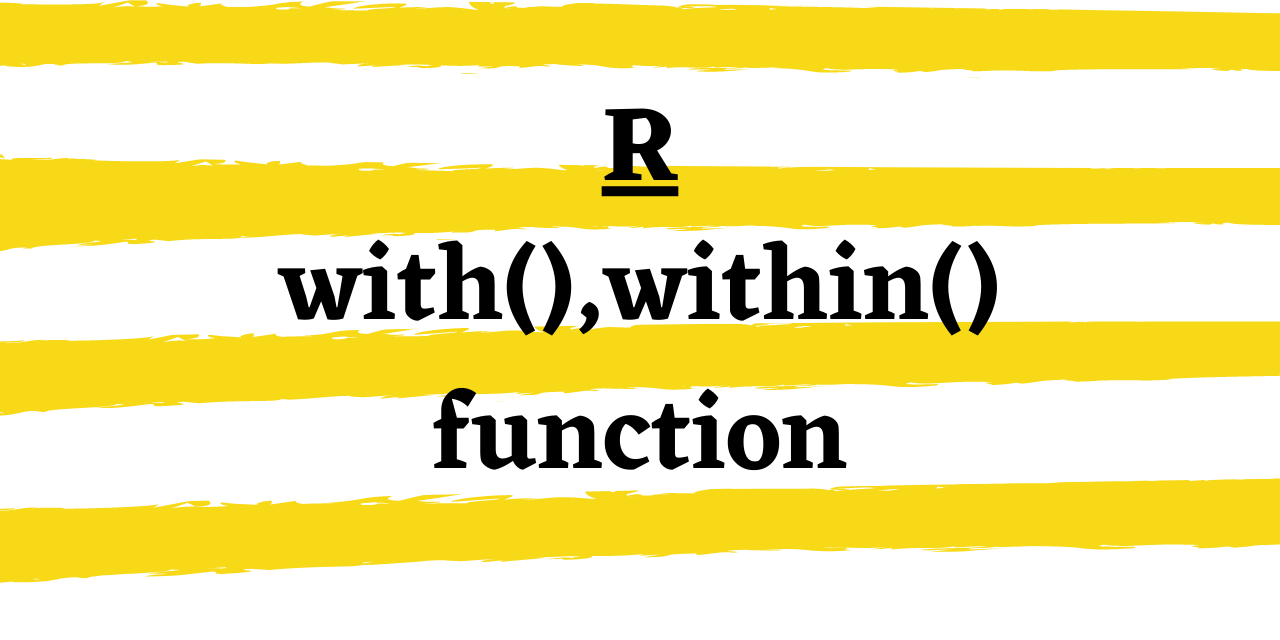# R with() and within() function - All you need to know!

Published on August 3, 2022By Safa Mulani
Developer and author at DigitalOcean.While we believe that this content benefits our community, we have not yet thoroughly reviewed it. If you have any suggestions for improvements, please let us know by clicking the “report an issue“ button at the bottom of the tutorial.

Hello, readers! In this article, we will be having a look at two closely related yet different functions of R programming - R with() and within() function, in detail. So, let us being!! :)

## 1. R with() function

We often come across situations wherein we feel the need to build customized/user-defined functions to conduct out a certain operation. With R with() function, we can operate on R expressions as well as the process of calling that function in a single go!

That is `with() function` enables us to evaluate an R expression within the function to be passed as an argument. It works on data frames only. That is why the outcome of the evaluation of the R expression is done with respect to the data frame passed to it as an argument.

Syntax:

``````with(data-frame, R expression)
``````

Example:

``````rm(list = ls())

Num <- c(100,100,100,100,100)
Cost <- c(1200,1300,1400,1500,1600)

data_A <- data.frame(Num,Cost,stringsAsFactors = FALSE)

with(data_A, Num*Cost)
with(data_A, Cost/Num)
``````

In the above example, we have calculated the expression ‘Num*Cost’ for the data frame data_A directly in the with() function.

After which, we have calculated the expression ‘Cost/Num’ within the function as well.

The reason behind having these two statements one after another is to highlight that the with() function does not alter the original data frame at any cost. It gives the output separately for every value associated with the columns of the data frame.

Output:

``````> with(data_A, Num*Cost)
 120000 130000 140000 150000 160000
> with(data_A, Cost/Num)
 12 13 14 15 16
``````

## 2. R within() function

Having read about with() function, now let us focus on it’s twin! Haha! Just joking! Though the names of the functions sound similar, the differ in the functioning.

R `within() function` calculates the outcome of the expression within itself but with a slight difference. It allows us to create a copy of the data frame and add a column that would eventually store the result of the R expression.

Syntax:

``````within(data frame, new-column <- R expression)
``````

Example:

``````rm(list = ls())

Num <- c(100,100,100,100,100)
Cost <- c(1200,1300,1400,1500,1600)

data_A <- data.frame(Num,Cost,stringsAsFactors = FALSE)

within(data_A, Product <- Num*Cost)
within(data_A, Q <- Cost/Num)

``````

Here, we have performed the evaluation of the same expressions that we had used for the with() function. But, here we have created a new column to store the outcome of the expression.

``````> within(data_A, Product <- Num*Cost)
Num Cost Product
1 100 1200  120000
2 100 1300  130000
3 100 1400  140000
4 100 1500  150000
5 100 1600  160000
> within(data_A, Q <- Cost/Num)
Num Cost  Q
1 100 1200 12
2 100 1300 13
3 100 1400 14
4 100 1500 15
5 100 1600 16
``````

## Conclusion

By this, we have come to the end of this topic. Feel free to comment below, if you come across any question.

For more such posts related to R, stay tuned and till then, Happy Learning!! :)Developer and author at DigitalOcean.